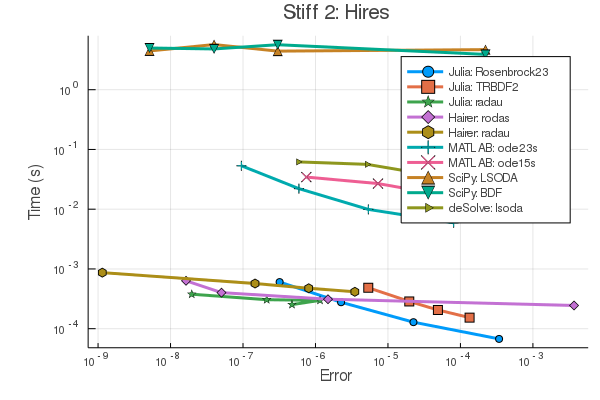# DifferentialEquations.jl v6.9.0: Automated Multi-GPU Implicit ODE Solving, SciPy/R Bindings

## Cluster Multi-GPU Support in DiffEqGPU

The DiffEqGPU automated GPU parallelism tools now support multiple GPUs. The README now shows that one can do things like:

# Setup processes with different CUDA devices
using Distributed

let gpuworkers = asyncmap(collect(zip(workers(), CUDAdrv.devices()))) do (p, d)
remotecall_wait(CUDAnative.device!, p, d)
p
end


to setup each individual process with a separate GPU, and then the standard usage of DiffEqGPU.jl:

function lorenz(du,u,p,t)
@inbounds begin
du = p*(u-u)
du = u*(p-u) - u
du = u*u - p*u
end
nothing
end

u0 = Float32[1.0;0.0;0.0]
tspan = (0.0f0,100.0f0)
p = (10.0f0,28.0f0,8/3f0)
prob = ODEProblem(lorenz,u0,tspan,p)
prob_func = (prob,i,repeat) -> remake(prob,p=rand(Float32,3).*p)
monteprob = EnsembleProblem(prob, prob_func = prob_func)
@time sol = solve(monteprob,Tsit5(),EnsembleGPUArray(),trajectories=100_000,
batch_size = 10_000, saveat=1.0f0)


will now make use of these GPUs per batch of trajectories. We can see effective parallel solving of over 100,000 ODEs all simultaneously using this approach on just a few compute nodes!

## SciPy and deSolve (R) (+Updated MATLAB) Common Interface Bindings for Ease of Translation

With the new SciPyDiffEq.jl, deSolveDiffEq.jl, and the update MATLABDiffEq.jl bindings, you can now solve common interface defined ordinary differential equations using the solver suites from Python, R, and MATLAB respectively. These libraries have been developed due to popular demand as a large influx of users from these communities who want to ensure that their Julia-translated models are correct. Now, one can install these solvers can double check their models with the original libraries to double check that the translation is correct.

To see this in action, the following solves the Lorenz equations with SciPy’s solve_ivp’s RK45, deSolve’s (R) lsoda wrapper, and MATLAB’s ode45:

using SciPyDiffEq, MATLABDiffEq, deSolveDiffEq

function lorenz(u,p,t)
du1 = 10.0(u-u)
du2 = u*(28.0-u) - u
du3 = u*u - (8/3)*u
[du1, du2, du3]
end
tspan = (0.0,10.0)
u0 = [1.0,0.0,0.0]
prob = ODEProblem(lorenz,u0,tspan)
sol = solve(prob,SciPyDiffEq.RK45())
sol = solve(prob,MATLABDiffEq.ode45())
sol = solve(prob,deSolveDiffEq.lsoda())


As an added bonus, this gives us a fairly simple way to track performance differences between the common ODE solver packages of each language. A new benchmark page is focused on cross language wrapper overhead and showcases the performance differences between these language’s differential equation suites on 4 ODE test problems (non-stiff and stiff). For example, on a system of 7 stiff ODEs, we see the following:which showcases the native Julia solvers as the fastest, benchmarking close to 50x faster than MATLAB, 100x faster than deSolve (R), and nearly 10,000x faster than SciPy. Thus, with these new tools, users can have a one line change to both ensure their models have translated correctly while understanding the true performance difference in their real-world context.

## Automated GPU-based Parameter Parallelism Support for Stiff ODEs and Event Handling

DiffEqGPU now supports stiff ODEs through implicit and Rosenbrock methods, and callbacks (both ContinuousCallback and DiscreteCallback) are allowed. To see this in action, one could for example do the following:

function lorenz(du,u,p,t)
@inbounds begin
du = p*(u-u)
du = u*(p-u) - u
du = u*u - p*u
end
nothing
end

u0 = Float32[1.0;0.0;0.0]
tspan = (0.0f0,100.0f0)
p = (10.0f0,28.0f0,8/3f0)

function lorenz_jac(J,u,p,t)
@inbounds begin
σ = p
ρ = p
β = p
x = u
y = u
z = u
J[1,1] = -σ
J[2,1] = ρ - z
J[3,1] = y
J[1,2] = σ
J[2,2] = -1
J[3,2] = x
J[1,3] = 0
J[2,3] = -x
J[3,3] = -β
end
nothing
end

nothing
end

prob_jac = ODEProblem(func,u0,tspan,p)
prob_func = (prob,i,repeat) -> remake(prob,p=rand(Float32,3).*p)
monteprob_jac = EnsembleProblem(prob_jac, prob_func = prob_func)

solve(monteprob_jac,Rodas5(linsolve=LinSolveGPUSplitFactorize()),EnsembleGPUArray(),dt=0.1,trajectories=10_000,saveat=1.0f0)
solve(monteprob_jac,TRBDF2(linsolve=LinSolveGPUSplitFactorize()),EnsembleGPUArray(),dt=0.1,trajectories=10_000,saveat=1.0f0)


This solves the Lorenz equations with Rosenbrock and implicit ODE solvers for 10,000 different parameters. On an example stiff ODE we’ve been testing (26 ODEs), a single RTX 2080 card was 5x faster than a multithreaded 16 core Xeon computer, meaning the time savings to do a parameter sweep with just one GPU can be tremendous, even (especially) on a stiff ODE.

## Stiff ODE Linear Solver Performance Improvements

Thanks to Yingbo Ma (@YingboMa), our implicit ODE solvers got a pretty major improvement in certain stiff ODEs which have fast oscillatory terms. Now it’s hard to find a stiff ODE benchmark where a native Julia method isn’t performing the best, except for super large systems where Newton-Krylov methods are used. Our next goal is to better enhance the performance of our Newton-Krylov support.

## More Precise Package Maintenance: Strict Versioning and Bounds

All of JuliaDiffEq now has upper bounds on its packages, along with CompatHelper installed so that every dependency change gets an automatic pull request and a notification to the JuliaDiffEq maintainers to inform us about changes in the wider Julia ecosystem. This should help us stay on top of all changes and keep the system stable.

# Next Directions

Here’s some things to look forward to:

• Automated matrix-free finite difference PDE operators
• Jacobian reuse efficiency in Rosenbrock-W methods
• Native Julia fully implicit ODE (DAE) solving in OrdinaryDiffEq.jl
• High Strong Order Methods for Non-Commutative Noise SDEs
• Stochastic delay differential equations